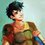# $\LaTeX$ Storage Note

To store $\LaTeX$ codes that might be useful later

Strictly not for chatting and trying $\LaTeX$ codes :)Note by Percy Jackson
2 months, 4 weeks ago

This discussion board is a place to discuss our Daily Challenges and the math and science related to those challenges. Explanations are more than just a solution — they should explain the steps and thinking strategies that you used to obtain the solution. Comments should further the discussion of math and science.

When posting on Brilliant:

• Use the emojis to react to an explanation, whether you're congratulating a job well done , or just really confused .
• Ask specific questions about the challenge or the steps in somebody's explanation. Well-posed questions can add a lot to the discussion, but posting "I don't understand!" doesn't help anyone.
• Try to contribute something new to the discussion, whether it is an extension, generalization or other idea related to the challenge.

MarkdownAppears as
*italics* or _italics_ italics
**bold** or __bold__ bold
- bulleted- list
• bulleted
• list
1. numbered2. list
1. numbered
2. list
Note: you must add a full line of space before and after lists for them to show up correctly
paragraph 1paragraph 2

paragraph 1

paragraph 2

[example link](https://brilliant.org)example link
> This is a quote
This is a quote
    # I indented these lines
# 4 spaces, and now they show
# up as a code block.

print "hello world"
# I indented these lines
# 4 spaces, and now they show
# up as a code block.

print "hello world"
MathAppears as
Remember to wrap math in $$ ... $$ or $ ... $ to ensure proper formatting.
2 \times 3 $2 \times 3$
2^{34} $2^{34}$
a_{i-1} $a_{i-1}$
\frac{2}{3} $\frac{2}{3}$
\sqrt{2} $\sqrt{2}$
\sum_{i=1}^3 $\sum_{i=1}^3$
\sin \theta $\sin \theta$
\boxed{123} $\boxed{123}$

Sort by:

so i have uncovered your tactic! now to give you a tste of ur own medicine :)

- 1 month, 3 weeks ago





































- 1 month, 3 weeks ago



- 1 month, 3 weeks ago

percy it seems like i have uncovered your method of creating the abyss thanks to the empty comment that pall mortom has made in my testing note i have concluded that that is the most probable way you made that abyss and i am going to test it here and develop ways of doing it without lag on old devices so just ignore any empty souls i put here

- 1 month, 3 weeks ago

LOL, you're so naive. YOur method is wrong, and don't dirty my storage note, do it in Latex Club if you like @Nathan Soliman

- 1 month, 3 weeks ago

well its just as good as urs

- 1 month, 2 weeks ago

no, mine is better

- 1 month, 2 weeks ago

how its nothing :)

- 1 month, 2 weeks ago

to test latex go here https://brilliant.org/discussions/thread/testing-19/

- 1 month, 4 weeks ago

\colorbox{white}{\large\color{white}\text{your text}} this is more for me to remember

- 2 months, 3 weeks ago

$\begin{array}{|l|l|l|l|l|l|l|} \hline\\[-7px]\hspace{15px}\href{#}{\textsf{Problem 1}}\hspace{15px} &\hspace{15px}\href{#}{\textsf{Problem 2}}\hspace{15px} &\hspace{15px}\href{#}{\textsf{Problem 3}}\hspace{15px} &\hspace{15px}\href{#}{\textsf{Problem 4}}\hspace{15px} &\hspace{15px}\href{#}{\textsf{Problem 5}}\hspace{15px} &\hspace{15px}\href{#}{\textsf{Problem 6}}\hspace{15px} &\hspace{15px}\href{#}{\textsf{Problem 7}}\hspace{15px}\\[3px]\hline\\[-7px] \hspace{15px}\href{#}{\textsf{Problem 8}}\hspace{15px} &\hspace{15px}\href{#}{\textsf{Problem 9}}\hspace{15px} &\hspace{15px}\href{#}{\textsf{Problem 10}}\hspace{15px} &\hspace{15px}\href{#}{\textsf{Problem 11}}\hspace{15px} &\hspace{15px}\href{#}{\textsf{Problem 12}}\hspace{15px} &\hspace{15px}\href{#}{\textsf{Problem 13}}\hspace{15px} &\hspace{15px}\href{#}{\textsf{Problem 14}}\hspace{15px}\\[7px] \hspace{15px}\href{#}{\textsf{Problem 15}}\hspace{15px} &\hspace{15px}\href{#}{\textsf{Problem 16}}\hspace{15px} &\hspace{15px}\href{#}{\textsf{Problem 17}}\hspace{15px} &\hspace{15px}\href{#}{\textsf{Problem 18}}\hspace{15px} &\hspace{15px}\href{#}{\textsf{Problem 19}}\hspace{15px} &\hspace{15px}\href{#}{\textsf{Problem 20}}\hspace{15px} &\hspace{15px}\href{#}{\textsf{Problem 21}}\hspace{15px}\\\hline \end{array}$

# Tables! (courtesy of Páll Márton)

- 2 months, 3 weeks ago

# $\overset{def}{=}$ - \overset{def}{=} - \overset command is useful to make def on your = signs :)

- 2 months, 4 weeks ago

# $\text{Rules -}$

$\text{1. Please don't use this note for chatting}$

$\text{2. This note is only to store your \LaTeX codes that you might need later, but take lot of space.}$

$\text{3. More rules will come later, but please follow the rules}$ :)

- 2 months, 4 weeks ago

@Percy Jackson, I forgot how to the equal sign with def over it. Can you help me?

- 2 months, 4 weeks ago

I posted in the Latex Club, please check there @Yajat Shamji.

- 2 months, 4 weeks ago

# $\text{Important facts}$

$\text{1. The rpm of a gear is inversely proportional to its number of teeth} \\ \text{2. The gears connected at the top of one another move with same speed.}$

# $\text{Visual explanation}$

<Picture Latex code here>

$\text{It is clear from the picture, that there is a/no problem in direction.} \\ \text{Hence, the system will/will not work.}$

# $\text{Casework}$

$\text{Without loss of generality, let the leftmost gear in some color rotate at 2 rpm.}$

# $\text{Up Setup}$

$\colorbox{silver}{\color{#333333}\text{Speed of Silver gear}} =\dfrac{50\times 2}{20}=5 \text{rpm}$

$\colorbox{#624F41}{\color{#FFFFFF}\text{Speed of brown gear}}=\dfrac{20\times 5}{10}=10 \text{ rpm}$

$\colorbox{silver}{\color{#333333}\text{Speed of Silver gear}} =\dfrac{50\times 2}{20}=5 \text{ rpm}$

$\colorbox{#624F41}{\color{#FFFFFF}\text{Speed of brown gear}}=\dfrac{20\times 5}{10}=10 \text{ rpm}$

$\colorbox{gold}{\color{#333333}\text{Speed of gold gear}} = \dfrac{20\times 5}{50}= 2\text{ rpm}$

$\colorbox{#815331}{\color{#FFFFFF}\text{Speed of Bronze gear}}=\dfrac{50\times 2}{25}=4 \text{ rpm}$

$\colorbox{#624F41}{\color{#FFFFFF}\text{Speed of inner brown gear}}=\dfrac{20\times 5}{25}=4 \text{ rpm}$

$\colorbox{#624F41}{\color{#FFFFFF}\text{Speed of brown gear}}=\dfrac{20\times 5}{25}=4 \text{ rpm}$

$\colorbox{#69047e}{\color{#FFFFFF}\text{Speed of Purple gear(A)}} =\dfrac{10\times 4}{20}=2\text{ rpm}$

# $\text{Down Setup}$

$\colorbox{#624F41}{\color{#FFFFFF}\text{Speed of brown gear}}=\dfrac{50\times 2}{10}=10 \text{ rpm}$

$\colorbox{silver}{\color{#333333}\text{Speed of silver gear}} = \dfrac{10\times 10}{20}= 5\text{ rpm}$

$\colorbox{gold}{\color{#333333}\text{Speed of gold gear}} = \dfrac{20\times 5}{50}= 2\text{ rpm}$

$\colorbox{#624F41}{\color{#FFFFFF}\text{Speed of inner brown gear}} \ \color{#333333}{=} \ \colorbox{gold}{\color{#333333}\text{Speed of gold gear}} = 2\text{ rpm}$

$\colorbox{gold}{\color{#333333}\text{Speed of gold gear}} =2\text{ rpm}$

$\colorbox{silver}{\color{#333333}\text{Speed of silver gear}} = \dfrac{20\times 2}{20}= 2\text{ rpm}$

$\colorbox{#624F41}{\color{#FFFFFF}\text{Speed of brown gear}}=\dfrac{20\times 2}{10}=4 \text{ rpm}$

$\colorbox{#3d99f6}{\color{#FFFFFF}\text{Speed of blue gear(B)}}=\dfrac{10\times 4}{20}=2 \text{ rpm}$

# $\text{Answer}$

$\large{\color{#69047e}\text{Speed of Purple gear(A)} = \color{#3d99f6}\text{Speed of Blue gear(B)}=\color{#333333}2\text{ rpm}}$

# Latex code for Gear Problems :)

- 2 months, 4 weeks ago